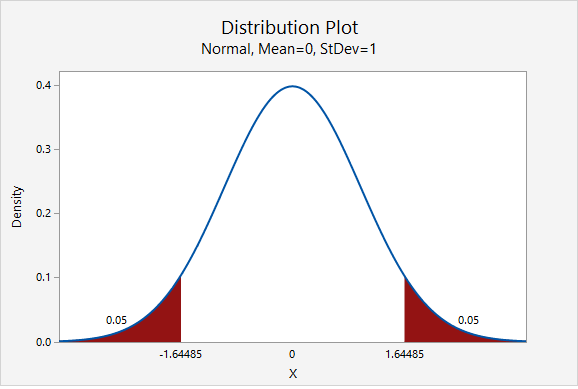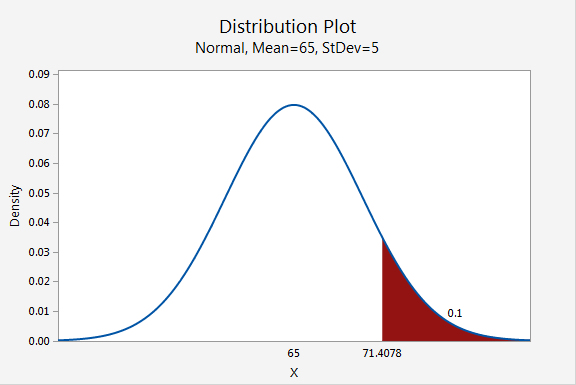# 7.3 - Minitab Express: Finding Values Given Proportions

7.3 - Minitab Express: Finding Values Given Proportions

## MinitabExpress – Finding the Middle 90% of a z Distribution

Scenario: What z scores separate the middle 90% of the standard normal distribution from the outer 10%?

We will construct a standard normal distribution with a mean of 1 and standard deviation of 0. We will find the points that separate the middle 0.90 from the outer 0.10. Note that the outer 0.10 will be equally split between the two tails:

Steps
1. On a PC: from the menu select STATISTICS > Distribution Plot
On a Mac: from the menu select Statistics > Probability Distributions > Distribution Plot
2. Select Display Probability (Note: The default is the standard normal distribution)
3. Select A specified probability
4. Select Equal tails
5. For Probability enter 0.10

This should result in the following output:The z scores that separate the middle 90% of the distribution from the outer 10% are ±1.64485

Video Walkthrough

Select your operating system below to see a step-by-step guide for this example.

## MinitabExpress – Finding the Top 10% of Vehicle Speeds

Scenario: Vehicle speeds at a highway location have a normal distribution with a mean of 65 mph and a standard deviation of 5 mph. What speed separates the top 10% of vehicles?

We will construct a normal distribution with a mean of 65 and standard deviation of 5. We will find the point that separates the to 0.10 from the bottom 0.90:

Steps
1. On a PC: from the menu select STATISTICS > Distribution Plot
On a Mac: from the menu select Statistics > Probability Distributions > Distribution Plot
2. Select Display Probability
3. For Distribution select Normal
4. For Mean enter 65
5. For Standard deviation enter 5
6. Select A specified probability
7. Select Right tail
8. For Probability enter 0.10

Note: You could also select Left tail and enter 0.90 as the probability.

This should result in the following output:The speed that separates the top 10% is 71.4078 mph.

Video Walkthrough

Select your operating system below to see a step-by-step guide for this example.

# 7.3.1 - Video Example: Middle 80% of the z Distribution

7.3.1 - Video Example: Middle 80% of the z Distribution

Question: What z scores separate the middle 80% of the z distribution from the outer 20%?

Steps
1. On a PC: from the menu select STATISTICS > Distribution Plot
On a Mac: from the menu select Statistics > Probability Distributions > Distribution Plot
2. Select Display Probability (Note: The default is the standard normal distribution)
3. Select A specified probability
4. Select Equal tails
5. For Probability enter 0.20
Video Walkthrough

# 7.3.2 - Video Example: Middle 50% SATM

7.3.2 - Video Example: Middle 50% SATM

Question: SAT-Math scores are normally distributed with a mean of 500 and standard deviation of 100. Find the SAT-Math scores that separate the middle 50% of the distribution from the outer 50% of the distribution.

Steps
1. On a PC: from the menu select STATISTICS > Distribution Plot
On a Mac: from the menu select Statistics > Probability Distributions > Distribution Plot
2. Select Display Probability
3. For Distribution select Normal
4. For Mean enter 500
5. For Standard deviation enter 100
6. Select A specified probability
7. Select Equal tails
8. For Probability enter 0.50
Video Walkthrough

# 7.3.3 - Video Example: Top 10% SATM

7.3.3 - Video Example: Top 10% SATM

Question: SAT-Math scores are normally distributed with a mean of 500 and standard deviation of 100. What score separates the top 10% from the bottom 90%?

Steps

1. On a PC: from the menu select STATISTICS > Distribution Plot
On a Mac: from the menu select Statistics > Probability Distributions > Distribution Plot
2. Select Display Probability
3. For Distribution select Normal
4. For Mean enter 500
5. For Standard deviation enter 100
6. Select A specified probability
7. Select Right tail
8. For Probability enter 0.10
Video Walkthrough

  Link ↥ Has Tooltip/Popover Toggleable Visibility